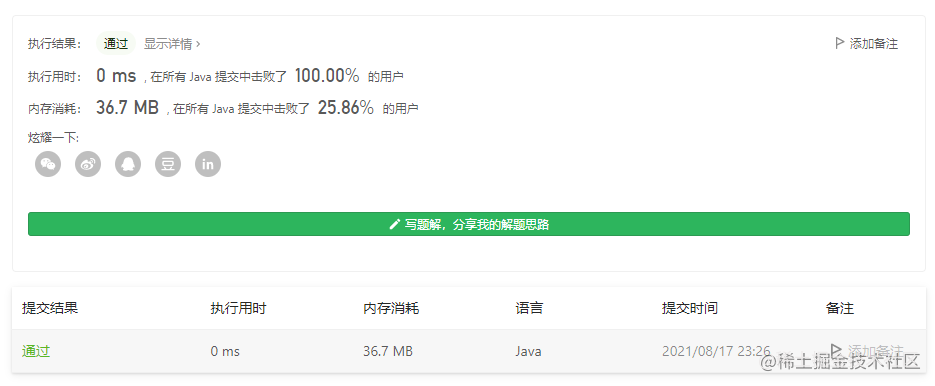# 每日算法篇（四）之学生出勤记录

## 题目

• 'A'：Absent，缺勤
• 'L'：Late，迟到
• 'P'：Present，到场

• 按 总出勤 计，学生缺勤（'A'）严格 少于两天。
• 学生 不会 存在 连续 3 天或 3 天以上的迟到（'L'）记录。
• 如果学生可以获得出勤奖励，返回 true ；否则，返回 false 。

## 思路+题解

• 超过或等于两次缺勤为失败
• 连续三次迟到也算失败，重点：连续；所以用一个初始化值去接收，并在每次不是L时归零

### 官方解析

``````class Solution {
public boolean checkRecord(String s) {
char[] a = s.toCharArray();
Integer maxA = 0;
Integer maxL = 0;
for(char q:a){
if(q == 'A'){
maxA++;
if(maxA == 2){
return false;
}
}
if(q == 'L'){
maxL++;
if(maxL>=3){
return false;
}
}else{
maxL =0;
}
}
return true;
}
}

## 总结

### 复杂度分析

• 时间复杂度：O(n)O(n)，其中 nn 是字符串 ss 的长度。需要遍历字符串 ss 一次。
• 空间复杂度：O(1)O(1)。